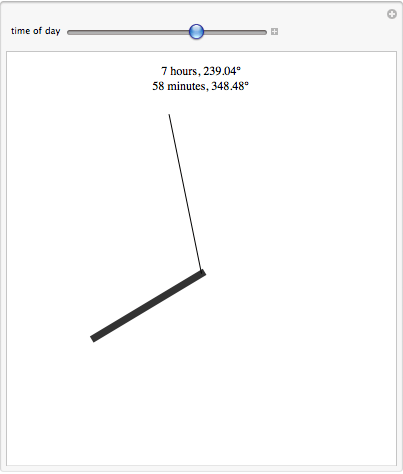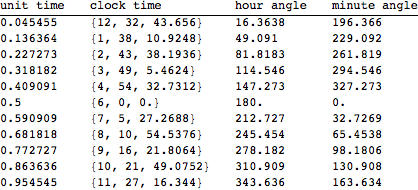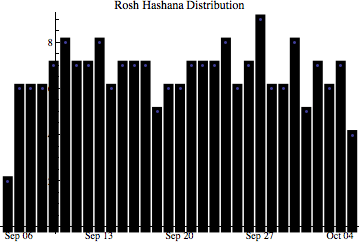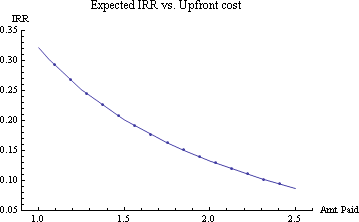# clock hands, part ii

I posted a request to mathgroup asking for guidance on why Solve[] and its brethren failed on the puzzle of the opposing clock hands. Two people have already responded with working versions from which we can draw the following conclusions,

1. We have to unwind the linedupedness[], unitTimeToHourAngle[], and unitTimeToMinuteAngle[] functions, and

2. We need to restrict the domain of the solution to the range from 0 to 1.

When we do this, we go from

`In[]:= Reduce[unstraightness[unitTime] == 0 && 0 < unitTime < 1]`
to
```In[]:= Solve[Abs[Abs[4320 Mod[unitTime, 1/12] - unitTime*360] - 180] == 0 && 0 < unitTime < 1, unitTime]```

which does indeed produce the right answer. There are other ways to get there too; unwinding and restricting the solution to Reals or Rationals will work, though we get some redundant solutions that way.

Wolfram tech support had an even nicer solution, pointing out that my wrapper function (unstraightness[]) could take any kind of input, while the internal functions (unitTimeToHourAngle[] and unitTimeToMinuteAngle[]) are restricted to real numbers. This means that when these functions are introduced into the Reduce function, unitTime is not defined as a number, so the function is never evaluated. The simplest solution is just to change the definition of the internal functions to

`In[]:= minuteAngle[unitTime_] := 4320 Mod[unitTime, 1/12]`
and
`In[]:= hourAngle[unitTime_] := unitTime*360 // N`

whereupon

```In[]:=Reduce[Abs[minuteAngle[unitTime] - hourAngle[unitTime]] == 0 && 0 < unitTime < 1]```

works like a charm.

# Opposing clock hands

I was recently sitting in a poorly produced play called That Hopey Changey Thing when, looking wistfully at my watch, I noticed that the hour hand and the minute hand were perfectly lined up, pointing in opposite directions. I stayed awake for the rest of the show in part thanks to the thought puzzle of how many times a day this happens, and how best to determine exactly when.

First, I found it useful to think of time in terms of a unit clock cycle — the hands on our theoretical clock run from 0 to 1, rather than to 12. The following Manipulate[] command draws a clock, allowing the user to set the unit time, and translating it into both conventional time and the angle of the minute and hour hands.

` In[]:= Manipulate[ Graphics[{{Opacity, Disk[{0, 0}, .9]}, {Opacity[.8], Thickness[.02], Line[{{0, 0}, .65 {Sin[2 Pi m], Cos[2 Pi m]}}]}, Line[{{0, 0}, .85 {Sin[12*2 Pi m], Cos[12*2 Pi m]}}]}, PlotLabel -> ToString[Floor[Mod[11 + m*12, 12] + 1]] <> " hours, " <> ToString[360.*m] <> "\[Degree]\n" <> ToString[Floor[Mod[m, 1/12]*720]] <> " minutes, " <> ToString[4320 Mod[m, 1/12]] <> "\[Degree]"], {{m, 0, "time of day"}, 0, 1}] `

The code when running looks likeThis was useful when debugging the formulae for the conversions; any error was immediately apparent.

Breaking out the conversions explicitly, we have

` In[]:= unitTimeToListTime[unitTime_Real] := Module[{hour, minute, seconds}, hour = Floor[Mod[11 + unitTime*12, 12] + 1]; minute = Floor[Mod[unitTime, 1/12]*720]; seconds = Mod[unitTime, 1/720]*60*720; {hour, minute, seconds} ] `
`In[]:= unitTimeToMinuteAngle[unitTime_Real] := 4320 Mod[unitTime, 1/12]`

and

`In[]:= unitTimeToHourAngle[unitTime_Real] := unitTime*360 // N`

These in hand, I naively thought the problem was as good as solved. I defined my problem in functional form,

`In[]:= unstraightness[unitTime_] := Abs[Abs[unitTimeToMinuteAngle[unitTime] - unitTimeToHourAngle[unitTime]] - 180]`

checked it with

`Plot[unstraightness[unitTime], {unitTime, 0, .999999}]`and we see as expected a periodic function. There are 11 solutions when “unstraightness” == 0 per day, which makes perfectly good sense when you think about it.

I thought I could determine these with `NSolve[unstraightness[unitTime] == 0, unitTime]`

but sadly, this yields nothing but error messages.
`NSolve::nsmet: This system cannot be solved with the methods available to NSolve. >>`

What to do? I decided on brute force. Slice the day into 1,000,000 pieces and identify the 11 times which produce the most opposed clock hands.

`In[]:= handangle = Table[{unitTime, Abs[unitTimeToMinuteAngle[unitTime] - unitTimeToHourAngle[unitTime]]}, {unitTime, 0, .999999, .000001}];`

`In[]:= closeFits = Select[handangle, Abs[#[] - 180] < .002 &];`

```In[]:= TableForm[ Map[{#, ToString[unitTimeToListTime[#]], unitTimeToHourAngle[#], unitTimeToMinuteAngle[#]} &, Transpose[closeFits][]], TableHeadings -> {None, {"unit time", "clock time", "hour angle", "minute angle"}}]```Ah-hah!

As looked to be the case from the graph, these solutions are clearly spaced exactly 1/11 of the clock apart from each other. This means that we can get a more perfect solution more quickly with

`In[]:= Table[ToString[unitTimeToListTime[i // N][]] <> ":" <> ToString[unitTimeToListTime[i // N][]] <> ":" <> ToString[unitTimeToListTime[i // N][]], {i, 1/22, .9999, 1/11}] // TableForm`

which returns

12:32:43.6364
1:38:10.9091
2:43:38.1818
3:49:5.45455
4:54:32.7273
6:00:00.
7:5:27.2727
8:10:54.5455
9:16:21.8182
10:21:49.0909
11:27:16.3636

Clearly, my bored glance at my watch had been at 8:10:54 pm.

One of the nice things about this solution method is that it easily generalizes to solve other “clock face” problems. For example, in a second we can determine that the hands of a clock are perfectly lined up, facing the same direction, at the following times:
12:00:00.
1:5:27.2727
2:10:54.5455
3:16:21.8182
4:21:49.0909
5:27:16.3636
6:32:43.6364
7:38:10.9091
8:43:38.1818
9:49:5.45455
10:54:32.7273

# recursion in Mathematica

I have been playing with creating pseudotexts via markov chains recently, and may post some code soon. Creating random text via markov chains has two steps — first you analyze the source material and make a tree that describes the likelihood of different words following each other, and second you create a random walk through that tree, respecting the relative probability of the different paths.

The first step is easily done via many different methods, but if you want a tree of arbitrary depth, it becomes most elegant to use recursion. This is no harder in Mathematica than any other language, and for the sake of any novices googling around for examples of using Mathematica recursively, note the following example —

If you want to compute factorials, in practice you would probably use Mathematica’s postfix operator, !
``` In:= 4! Out= 24 ```

``` You could program your own version about a thousand different ways. I find this to be natural: In:= functionalFactorial[x_] := Apply[Times, Range[x]] In:= functionalFactorial Out= 24 And if you wanted a recursive version, you could do In:= recursiveFactorial[x_] := If[x == 1, 1, x*recursiveFactorial[x - 1]] In:= recursiveFactorial Out= 24 As in any recursive code, you need a test in the inner loop that defines at least one stopping point (in this case when we're multiplying by 1). Without that branch, all recursions would continue indefinitely. Either of the user-defined functions above could be compiled for additional speed. ```
``` This entry was posted in mathematica on October 9, 2010 by stern. ```
``` Jewish New Year Distribution I was talking to somebody the other day who was pleased because his birthday (September 9) landed on the Jewish New Year. He made the dubious claim that this happened something like 17% of the time. For a quick check, I used the JewishNewYear[] function built in to Mathematica’s Calendar package. It is good from 1900 through 2100. Needs["Calendar`"] In[]:= Length[Select[ Map[JewishNewYear[#] &, Range[1900, 2100]], #[] == 9 && #[] == 9 &]] Out[]= 7 7/Length[Range[1900, 2100]] == .0348, so over this 201 year time span, his birthday would have landed on Rosh Hashana a bit under 3.5% of the time. Curious about how the holiday distributed, I generated a table of frequencies (all displayed as though they were 2010 dates so we can use the built-in DateListPlot[] function). In[]:= dateFreq = Map[{#[], Length[#]} &, Gather[Sort[ Map[{2010, JewishNewYear[#][], JewishNewYear[#][]} &, Range[1900, 2100]], AbsoluteTime[#1] <= AbsoluteTime[#2] &]]]; In[]:= DateListPlot[dateFreq, Filling -> Axis, FillingStyle -> {Automatic, {Black, AbsoluteThickness}}, GridLines -> None, Frame -> False, Axes -> True, AxesStyle -> Black, AxesOrigin -> {Automatic, 0}, PlotRange -> All, PlotLabel -> "Rosh Hashana Distribution"]Note the nested use of Length[], Gather[], Sort[]. That is often a powerful combination when using Mathematica to analyze the frequencies of events or patterns in data. In this case we do not have a uniform distribution — it clearly fades at the edges, but it’s not normally distributed either. Figuring out whether or not there is a meaningful pattern here is beyond my statistical skills. This entry was posted in mathematica on September 16, 2010 by stern. reversing the x-axis in a Mathematica graph Sometimes you have data in which the dependent variable decreases as the independent variable increases, and for reasons of clarity in illustrating it, you want to flip the x-axis and show an increasing line (or vice versa). For example, sometimes you haveand you want to display it asUnfortunately, Mathematica’s default behavior is to show both axes growing as you go up and to the right, and there is no simple option to change that. You can achieve this however by transforming your data by hand so that it is displaying the desired way, and then setting the “Ticks” variable of the plotting function to display the Ticks in reverse order. You can’t just say “reverse,” you have to create a list of the new tick labels and where you want them to go. This is generally done with a call to the Table[] command. I more commonly flip the X axis than the Y, and given data in {{x1,y1},{x2,y2} . . . {xn,yn}} format, the following code makes it very easy to do. xFlipper[listofXYpairs_] := Map[{Last[listofXYpairs][] + First[listofXYpairs][], 0} + {-1, 1}*# &, listofXYpairs] Options[xFlippedTicks] = {numPoints -> 10, digits -> "All"}; xFlippedTicks[listofXYpairs_, OptionsPattern[]] := Table[{Last[listofXYpairs][] + First[listofXYpairs][] - i, If[OptionValue[digits] == "All", i, Round[i, 10^-OptionValue[digits] // N]]}, {i, First[listofXYpairs][], Last[listofXYpairs][], ( Last[listofXYpairs][] - First[listofXYpairs][])/ OptionValue[numPoints]-1}] The numpoints variable sets how many points to show on the X axis. The digits variable can reduce the number of the digits shown in the tickmarks if needed to make the graph more useful or attractive. The first graph above was produced with ListPlot[returnPairs, PlotLabel -> "Expected IRR vs. Upfront cost", AxesLabel -> {"Amt Paid", "IRR"}, Joined -> True, Mesh -> True] Given these two functions, we can transform it to the second graph above with ListPlot[xFlipper[returnPairs], PlotLabel -> "Expected IRR vs. Upfront cost", Ticks -> {xFlippedTicks[returnPairs, numPoints -> 5], Automatic}, AxesLabel -> {"Amt Paid", "IRR"}, Joined -> True, Mesh -> True] (The “automatic” in the Tick specification tells Mathematica to continue to handle the Y axis automatically.) This entry was posted in mathematica on August 27, 2010 by stern. Post navigation ← Older posts Newer posts → ```